# differential calculus

Also found in: Dictionary, Thesaurus, Medical, Wikipedia.
Related to differential calculus: integral calculus

## differential calculus:

see calculuscalculus,
branch of mathematics that studies continuously changing quantities. The calculus is characterized by the use of infinite processes, involving passage to a limit—the notion of tending toward, or approaching, an ultimate value.
.
The following article is from The Great Soviet Encyclopedia (1979). It might be outdated or ideologically biased.

## Differential Calculus

a branch of mathematics that studies derivatives and differentials of functions and their use in the investigation of functions.

The development of differential calculus into an independent mathematical discipline is associated with the names of I. Newton and G. von Leibniz (the second half of the 17th century), who formulated the basic propositions of differential calculus and clearly indicated the mutually inverse nature of the operations of differentiation and integration. Since that time differential calculus has developed with integral calculus. The (differential and integral) calculus form the main part of mathematical analysis. The creation of the calculus launched a new era in the development of mathematics. It resulted in the appearance of a number of mathematical disciplines: the theory of series, the theory of differential equations, differential geometry, and the calculus of variations. The methods of mathematical analysis have found application in all divisions of mathematics. The number of applications of mathematics to problems of natural science and technology has expanded immeasurably. “Only differential calculus makes it possible for natural science to depict mathematically not only states, but also processes: motion” (F. Engels; see K. Marx and F. Engels, Soch., 2nd ed., vol. 20, p. 587).

Differential calculus is based on the following fundamental concepts of mathematics: real numbers (the number line), function, limit, and continuity. All these concepts have become crystallized and have obtained their present content in the course of the development and substantiation of the calculus. The basic idea of the differential calculus consists in studying functions locally. To be more precise, the differential calculus supplies the apparatus for studying functions whose behavior in a sufficiently small neighborhood of each point is close to the behavior of a linear function or polynomial. The central concepts of the differential calculus, the derivative and the differential, serve as such an apparatus. The concept of a derivative developed out of a large number of problems of natural science and mathematics, all of which reduced to calculating limits of the same type. The most important of these problems were the determination of the velocity of rectilinear motion of a point and the construction of a tangent to a curve. The concept of a differential is the mathematical expression of the proximity of a function to a linear function in a small neighborhood of the point being examined. In contrast to a derivative, the concept of a differential is easily carried over to mappings of one Euclidian space into another and to mappings of arbitrary normed vector spaces and is one of the principal concepts of modern nonlinear functional analysis.

The derivative. Consider the problem of the determination of the velocity of a material point moving in a straight line. If the motion is uniform, then the distance traversed by the point is proportional to time elapsed. The velocity of such a motion can be defined as the (directed) distance traversed by the point during one time unit or as the quotient of the (directed) distance and the corresponding time interval. If the motion is not uniform, then the distances corresponding to equal time intervals will, in general, be different. An example of nonuniform motion is the motion of a body falling freely in a vacuum. The law of motion of such a body is expressed by the formula s = gt2/2, where s is the distance covered from the beginning of the fall (in meters), t is the time of the fall (in seconds), and g is a constant, the acceleration of free fall (g ≈ 9.81 m/sec2). After the first second, the body will cover approximately 4.9 m, after the second, approximately 14.7 m, and after the tenth, approximately 93.2 m, that is, the fall does not proceed uniformly. Therefore, the aforementioned definition of velocity is not applicable here. In this case we consider the average velocity of motion over some time interval after (or before) the fixed moment t. The average velocity is defined as the quotient of the distance traversed by the time elapsed. This average velocity depends not only on the moment t but also on the selection of the time interval. In our example, the average velocity of fall over the time interval from t to t + Δr is equal to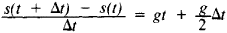If the time interval Δt tends to zero, then this expression tends to the number gt, which is called the velocity of the motion at the time t. Thus, the velocity of motion at any moment of time is defined as the limit of the average velocity as the time interval tends to zero.

In general, these calculations must be made for any moment of time t, for the time interval from t to t + Δt, and for the law of motion given by the formula s = f(t). Then the average velocity of motion over the interval of time from t to t + Δt is given by the formula Δst, where Δs =f(t + Δt) - f(t), and the velocity of motion at time t is equal to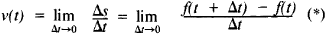The main advantage of the concept of velocity at a given moment, or instantaneous velocity, over average velocity is that it, like the law of motion, is a function of time t and not a function of the interval (t, t + Δt). On the other hand, the instantaneous velocity is somewhat of an abstraction, since it is the average velocity and not the instantaneous velocity that can be measured directly.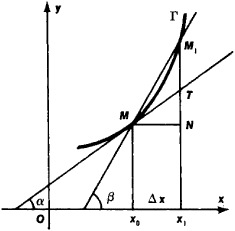Figure 1

The problem (see Figure 1) of constructing a tangent to a plane curve at some point M on the curve also leads to an expression of type (*). Let the curve F be the graph of the function y =f(x). The position of the tangent will be determined if we know its slope, that is, the tangent of angle a which is formed by the tangent with the Ox axis. Let us designate by XG the abscissa of the point M and by x1=x0+Δx the abscissa of the point M1. The slope of the secant MM1 is equal to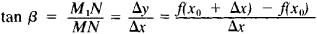where Δy = M1N = f(x0 + Δx) - f(x0) is the increment of the function on the segment [x0, x1]. By defining the tangent at the point M as the limiting position of the secant MM1 as x1 tends to x0, we obtain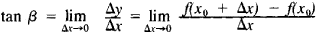If we ignore the mechanical or geometric content of the cited examples and focus instead on their common means of solution, we arrive at the concept of a derivative. We define the derivative y’ of the function y =f(x) at point x as the limit (if it exists) of the ratio of the increment of the function to the increment of the argument as the latter approaches zero, that is,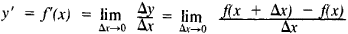In addition to the concepts already examined, a number of important concepts of natural science are defined with the aid of the derivative. For example, the intensity of a current is defined as the limit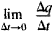where Δq is the positive electric charge that flows across a section of a circuit in time Δt. The speed of a chemical reaction is defined as the limit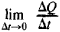where ΔQ is the change in the quantity of a substance over time Δt. In general, the derivative with respect to time is a measure of the speed of a process, which is applicable to the most diverse physical quantities.

The derivative of a function y = f(x) is denoted byf(x), y’, dy/dx, dfldy, orDf(x). If the function y = f(x) has a derivative at a point x0, then the function is defined at x0 and in some neighborhood of x0 and is continuous at x0. However, the opposite conclusion is incorrect. For example, the function y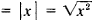, which is continuous at every point and whose graph consists of two rays issuing from the origin and forming angles of 45° and 135° with the Ox axis, does not have a derivative at x0, since the ratio Δy/Δx does not have a limit as Δx→0: when Δx > 0, this ratio is equal to + 1, and when Δx < 0, it is equal to - 1. Moreover, there are continuous functions that have no derivative at any point.

The operation of finding a derivative is called differentiation. In the class of functions that have a derivative, this operation is linear. Table 1 gives some rules of differentiation; in particular, it shows that the derivative of every elementary function is again an elementary function.

If the derivative f’(x) in turn has a derivative, then it is called the second derivative of the function y = f(x) and is designated as y”, f(x),d2y/dx2,d2f/dx2, or D2f(x). For a point moving in a straight line, the second derivative characterizes its acceleration.

Derivatives of a higher (integral) order are defined in an analogous manner. The derivative of order n is denoted by yn,fn(x),dny/dxn,dnf/dxn, or Dnf(x)

The differential. The function y = f(x), whose domain of definition contains some neighborhood of the point x0, is said to be differentiable at x0 if its increment

Δy - f(x0 + Δx) - f(x0)

can be written in the form

Δy = AΔx + αΔx

where A = A(x0), α = α(x, x0)→0 as x→x0. In that case the expression Δx is called the differential of the functionf(x) at x0 and is designated by dy or df(x0). Geometrically, the differential (for a fixed value of x0 and a changing increment Δx) represents the increment of the ordinate of the tangent, that is, the segment NT (see Figure 1). The differential dy is a function both of the point x0 and of the increment Δx. We say that the differential is the linear part of the increment of a function, meaning by this that for a fixed x0, dy is a linear function of Δx and that the difference Δy - dy is infinitesimal relative to Δx. For the function f(x) Ξx we have dx = Δx, that is, the differential of the independent variable coincides with its increment. Therefore, we usually write dy = Adx. There is a close relationship between the differential of a function and its derivative. In order for the function of one variable y = f(x) to have a differential at a point x0, it is necessary and sufficient that it have at this point the (finite) derivative f’(x0), and the equality dy =f(x0)dx is valid. The intuitive meaning of this supposition is that the tangent to the curve y = f(x) at the point with abscissa x0 is not only the limiting position of the secant but also the straight line which in an infinitesimal neighborhood of x0 is closer to the curve than any other line. Thus, A(x0) = f(xQ) always holds. The notation dy/dx can be thought of not only as the designation for the derivative/’ (x) but also as the quotient of the differentials of the dependent and independent variables. In view of the equality dy = f (x0)dx, the rules for finding differentials follow directly from the corresponding rules for finding derivatives.

Differentials of higher orders are also considered. We often use differentials to make approximate calculations of the values of functions and to estimate errors in calculations. Suppose for example, that we wish to calculate the value of a function f(x) at a point x given f(x0) and f(x0). By replacing the increment of the function with its differential we obtain the approximate equality

f(x1)≈f(x0) + df(x0) = f(x0)f(x0)(x1 - x0)

The error in this equality is approximately equal to half of the second differential of the function, that is,

½d2 = ½f(x0)(x1 - x0)2

Applications. In the differential calculus relationships are established between the properties of a function and its derivatives (or differentials). These relationships are expressed by the fundamental theorems of the differential calculus, which include Rolle’s theorem; the Lagrange formula [main statement of the mean value theorem] f(a) -f(b) =f(c)(b -a), where a < c <b; and Taylor’s formula.

These propositions make it possible to conduct a detailed study of the behavior of sufficiently smooth functions (that is, functions having derivatives of a sufficiently high order) using the methods of differential calculus. In this way we are able to study the degree of smoothness, the convexity and concavity of functions, the increase and decrease of functions, and the extrema of functions; to find their asymptotes and points of inflection; to calculate the curvature of a curve; and to examine the nature of its singular points. For example, the condition f’(X) > 0 entails a (strict) increase in the function y =f(x), while the condition f”(x) > 0 entails its (strict) convexity. The maximum and minimum points of a differentiable function, which are interior points of its domain of definition, are among the roots of the equation/’ (x)= 0.

The study of functions with the aid of derivatives constitutes the main application of the differential calculus. Moreover, the differential calculus makes it possible to calculate different types of limits of functions, in particular, limits of the type 0/0 and ∞/∞. The differential calculus is especially convenient for the study of elementary functions, since their derivatives can be written down explicitly.

Differential calculus of functions of many variables. The methods of the differential calculus are used in the study of functions of several variables. For a function of two independent variables z = f(x,y), the partial derivative of this function with respect to x is the derivative of this function with respect to x at constant y. This partial derivative is designated by zx’fx’(x,y),∂z/∂x, or ∂f(x,y)∂/x, so that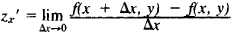The partial derivative of z with respect to y is defined and designated in an analogous manner. The quantity

Δz = f(x + Δx,y + Δy) - f(x,y)

is called the increment of the function z = f(x, y). If it can be represented in the form

Δz = AΔx + BΔy + α

where α is an infinitesimal of order higher than the distance between the points (x,y) and (x + Δx, y + Δy), then we say that the function z = f(x, f(x, y) is differentiable. The expression A Δx + BΔy forms the differential dz of the function z = f(x, y); in this case, A= zx’ and B = zy. Instead of Δx and Δy we usually write dx and dy, so that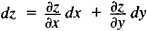Geometrically, the differentiability of a function of two variables implies that its graph has a tangent plane, and the differential is the increment of the z-coordinate of the tangent plane when the independent variables increase by dx and dy. For functions of two variables, the concept of a differential is considerably more important and natural than the concept of partial derivative. In contrast to functions of one variable, the existence of both partial derivatives of the first order for a function of two variables still does not guarantee its differentiability. However, if the partial derivatives are also continuous, then the function is differentiable.

Partial derivative of higher orders are defined in an analogous manner. The partial derivatives ∂2f/∂x∂y and ∂2f/∂y∂x are called mixed. If the mixed partial derivatives are continuous, then they are equal. All these definitions and designations apply to the case of functions of more than two variables.

History. The ancient Greek mathematicians could determine tangents to particular curves and could find the maxima and minima of particular variable quantities. For example, they found methods of constructing tangents to conic sections and some other curves. However, the methods worked out by the ancient mathematicians were applicable only to very special cases and were far from the ideas of the differential calculus.

We might say that the differential calculus was created as an independent branch of mathematics when it was understood that the indicated special problems together with a number of other problems (in particular, the problem of determining instantaneous velocity) were solved with the aid of the same mathematical apparatus—derivatives and differentials. This understanding was achieved by I. Newton and G. von Leibniz.

About 1666, Newton worked out the method of fluxions. Newton formulated the basic problems in terms of mechanics: (1) the determination of the velocity of motion using the known dependence of distance traversed on time and (2) the determination of the distance traversed in a given time given the known velocity of the motion. Newton called the continuous variable the fluent and its velocity the fluxion. Thus for Newton, the main concepts were the derivative (the fluxion) and the indefinite integral, or antiderivative (the fluent). He attempted to substantiate the method of fluxions by using the theory of limits, although the latter was only outlined by him.

In the mid-1670’s, Leibniz worked out a very convenient algorithm of the differential calculus. For Leibniz the basic concepts were the differential as an infinitesimal increment of a variable and the definite integral as the sum of an infinitely large number of differentials. To Leibniz belongs the credit for designating the differential dx and the integral ∫ dx , for a number of rules of differentiation, for a convenient and flexible notation, and, finally, for the very term “differential calculus.” The further development of the differential calculus initially proceeded along the path outlined by Leibniz; an important role was played at this stage by the brothers Jakob and Johann Bernoulli and B. Taylor.

The next stage in the development of the differential calculus was due to L. Euler and J. Lagrange (18th century). Euler for the first time set it forth as an analytic discipline, independent of geometry and mechanics. He again advanced the derivative as the basic concept of the differential calculus. Lagrange attempted to construct the differential calculus algebraically by using the expansion of functions in power series. He is responsible, in particular, for introducing the term “derivative” and the designations y’ and/’ (x). At the beginning of the 19th century, the problem of substantiating the differential calculus on the basis of the theory of limits was satisfactorily resolved. This was accomplished primarily as a result of the work of A. Cauchy, B. Bolzano, and K. Gauss. A more thorough analysis of the fundamental concepts of the differential calculus was connected with the development of set theory and the theory of functions of a real variable at the end of the 19th and the beginning of the 20th century.

### REFERENCES

History
Wileitner, H. Istoriia matematiki ot Dekarta do serediny 19 stoletiia, 2nd ed. Moscow, 1966. (Translated from German.)
Struik, D. Ia. Kratkii ocherk istorii matematiki, 2nd ed. Moscow, 1969. (Translated from German.)
Cantor, M. Vorlesungen tüber Geschichte der Mathematik, 2nd ed., vols. 3–4. Leipzig-Berlin, 1901–24.
Works of the founders and classics of differential calculus
Newton, I. Matematicheskie raboty. Moscow-Leningrad, 1937. (Translated from Latin.)
Leibniz, G. von. “Izbrannye otryvki iz matematicheskikh sochinenii” (translated from Latin). Uspekhi matematicheskikh nauk, 1948, vol. 3, issue 1.
L’Hospital, G. F. de. Analiz beskonechno malykh. Moscow-Leningrad, 1935. (Translated from French.)
Euler, L. Vvedenie v analiz beskonechnykh, 2nd ed., vol. 1. Moscow, 1961. (Translated from Latin.)
Euler, L. Differentsial’noe ischislenie. Moscow-Leningrad, 1949. (Translated from Latin.)
Cauchy, A. L. Kratkoe izlozhenie urokov o differentsial’ nom i integral’nom ischislenii. St. Petersburg, 1831. (Translated from French.)
Cauchy, A. L. Algebraicheskii analiz. Leipzig, 1864. (Translated from French.)
Textbooks and study guides on differential calculus
Khinchin, A. Ia. Kratkii kurs matematicheskogo analiza, 3rd ed. Moscow, 1957.
Khinchin, A. Ia. Vosem’ lektsii po matematicheskomu analizu, 3rd ed. Moscow-Leningrad, 1948.
Smirnov, V. I. Kurs vysshei matematiki, 22nd ed., vol. 1. Moscow, 1967.
Fikhtengol’ts, G. M. Kurs differentsial’nogo i integralnogo ischisleniia, 7th ed., vol. 1. Moscow, 1969.
La Vallée-Poussin, C. J. de. Kurs analiza beskonechno malykh, vol. 1. Leningrad-Moscow, 1933. (Translated from French.)
Courant, R. Kurs differentsial’nogo i integral’nogo ischisleniia. 4th ed., vol. 1. Moscow, 1967. (Translated from German and English.)
Banach, S. Differentsial’noe i integral’noe ischislenie, 2nd ed. Moscow, 1966. (Translated from Polish.)
Rudin, W. Osnovy matematicheskogo analiza. Moscow, 1966. (Translated from English.)

Edited by S. B. STECHKIN

## differential calculus

[‚dif·ə′ren·chəl ′kal·kyə·ləs]
(mathematics)
The study of the manner in which the value of a function changes as one changes the value of the independent variable; includes maximum-minimum problems and expansion of functions into Taylor series.
McGraw-Hill Dictionary of Scientific & Technical Terms, 6E, Copyright © 2003 by The McGraw-Hill Companies, Inc.

## differential calculus

the branch of calculus concerned with the study, evaluation, and use of derivatives and differentials
Collins Discovery Encyclopedia, 1st edition © HarperCollins Publishers 2005
References in periodicals archive ?
A "fantastically beautiful and powerful application of the differential calculus [that is] possibly the most useful" (p.
As a result, the differential calculus technique has been extended to [Z.sub.2]-graded commutative rings [5,8,9].
Let us mention that d : U [right arrow] [[OMEGA].sup.1.sub.q] is a first-order differential calculus over the algebra U.
Two classes of 39 students each from the College of Computer Studies, De La Salle University, who were enrolled in ANMATH1 (Differential Calculus) during the third term, SY 2005-2006 served as respondents of the study.
This new formalism has, for the first time, provided an irreversible description of thermodynamics via an irreversible differential calculus together with the related mathematics.
The late-seventeenth century was clouded with speculation as to who was the first developer of differential calculus, the German mathematician Gottfried Wilhelm Leibnitz or the English mathematician Isaac Newton.
Around 40pc of the questions on that paper, such as differential calculus, would these days be taught at AS level.
He married and had five children, but little is known about his life, though he is regarded as the founder of probability theory, the inventor of the differential calculus and the co-founder with Rene Descartes of modern number theory.
Gen: I'm very good at integral and differential calculus,
During that period, he began to invent a set of mathematical techniques, including what we call the binomial theorem and differential calculus. He did a set of optical experiments that demonstrated that white light is composed of a mixture of all colors of the rainbow.
A historian, shall we say a characteristic reader of the Canadian journal of History, would be totally baffled by the author's careful and subtle discussion of the similarities and differences between the treatments of the differential calculus by Leonhard Euler (1707-83) and Joseph-Louis Lagrange (1736-1813), with particular reference to the foundations of the subject.
One more thing: formulating and asking questions related to high order abstractions, principles, policies, opinions, observations, feelings, and anything you can think of, can be considered as a breakdown and application of the principles of the differential calculus. And I leave you with a definition of the calculus which I have found useful and which has expanded my awareness of myself and my environmental structures - the study of a continuous function by following its history by indefinitely small steps.

Site: Follow: Share:
Open / Close